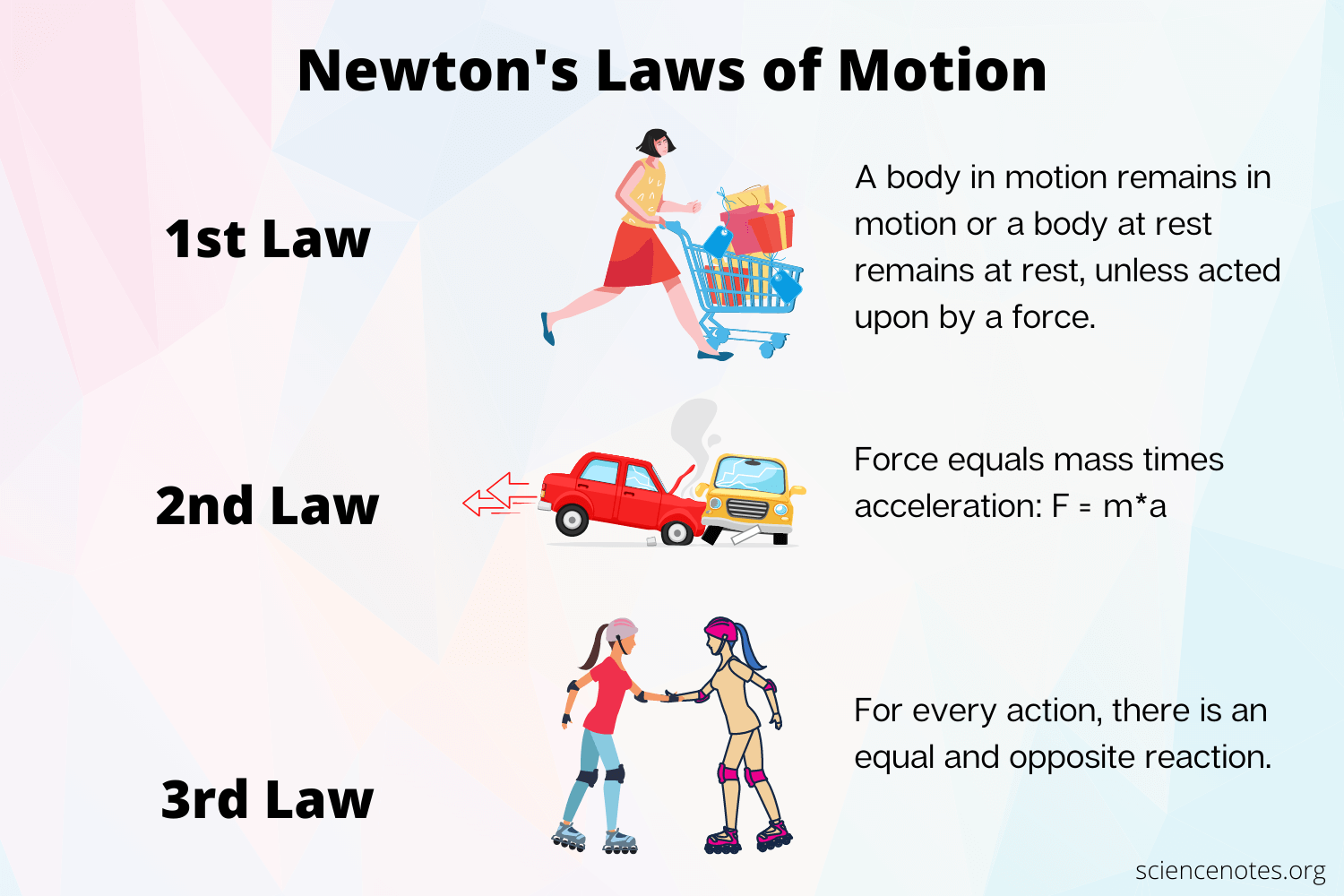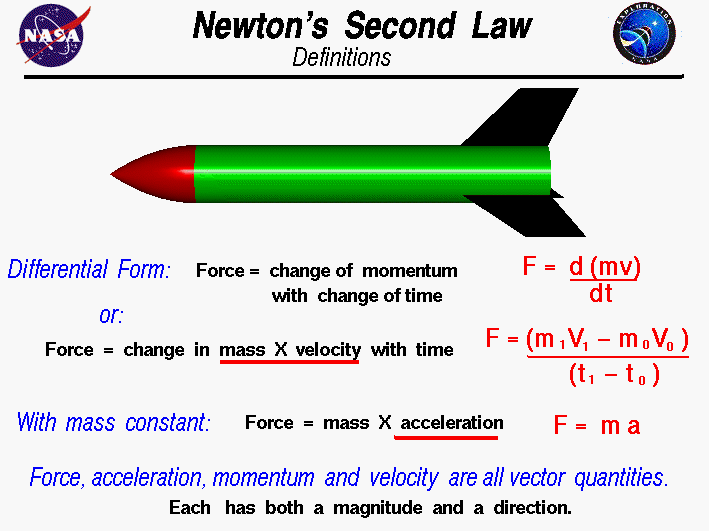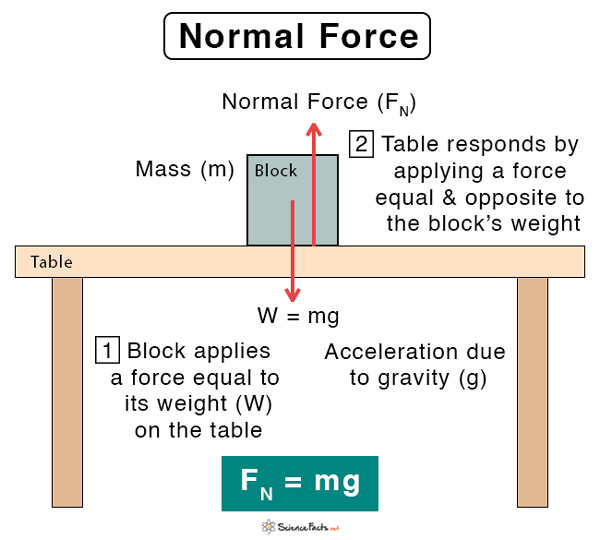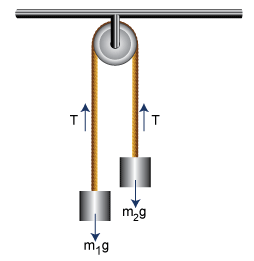# CBSE Class 11 Physics Chapter 5 Revision Notes

Chapter 5: Laws of Motion Revision Notes

• Dynamics is a branch of physics that studies the motion of a body while taking into account the cause, i.e. the force that causes the motion.
• Force: A force is an external force that produces or tries to produce motion in a still body, stops or tries to stop a moving body, or changes or tries to change the direction of motion of a moving body.
• Inertia is the inherent property of a body that prevents it from changing its state of motion. The inertia increases as the body weight increases, and the inertia decreases as the body weight decreases.
• The law of inertia states that a body’s state of rest, uniform motion (i.e., a motion with constant velocity), or motion direction cannot be changed by itself.

## Newton’s Laws of MotionSource

Law 1:

• Unless an external force is applied to a body, it will remain at rest or continue to move at a uniform velocity.
• The ‘Law of Inertia’ is another name for the first law of motion. It establishes the concepts of inertia, force, and inertial frame of reference.
• To describe and understand particle motion, there is always a need for a ‘frame of reference,’ and the simplest ‘frame of reference’ used are known as inertial frames.
• A referent frame, e, is known as an inertial frame because all accelerations of any particle within it are caused by the action of ‘real forces’ on that particle.
• The frame of reference used when discussing accelerations caused by “fictitious” or “pseudo” forces is non-inertial.

Law 2:

• When an external force is applied to a body of constant mass, it causes an acceleration that is proportional to the force and inversely proportional to the mass of the body.Source

Law 3:

“There is an equal and opposite reaction force to every action.” When a body A applies a force to another body B, B responds by applying an equal and opposite force to A.

Linear Momentum:

The product of a body’s mass and velocity is defined as the linear momentum of the body.

Impulse:

Impulsive forces are those that act for a short period of time. The product of force and the short time interval over which it acts is defined as the impulse.

Law of Conservation of Momentum:

An isolated system of particles’ total momentum is conserved. In other words, the system’s total momentum remains constant when no external force is applied.

Commonly Used Forces

(i) Weight of a Body: It’s the force that pulls a body towards the center of the earth. The weight of the body is Mg in a vertically downward direction if M is the mass of the body and g is the acceleration due to gravity.

(ii) Normal Force: When two bodies come into contact, a contact force is created, which is normal to the plane of contact if the surface is smooth. This force is referred to as normal force.Source

(iii) String tension: Assume a block is suspended from a string. The block’s weight is acting vertically downward but it is not moving, so it is balanced by a force due to the string. ‘Tension in string’ is the name given to this force. In a stretched string, tension is a force. Its path is along the string, away from the subject’s body.

Simple Pulley

• Consider two bodies with masses m1 and m2 tied together at the ends of an inextensible string that passes through a light, friction-free pulley.Source

]]>